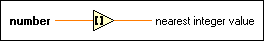# Round To Nearest Function

## LabVIEW 2018 Help

Edition Date: March 2018
Part Number: 371361R-01
View Product InfoLabVIEW 2016 HelpLabVIEW 2017 HelpLabVIEW 2018 HelpLabVIEW 2019 HelpLabVIEW 2020 Help

Owning Palette: Numeric Functions

Requires: Base Development System

Rounds the input to the nearest integer. If the value of the input is midway between two integers, the function returns the nearest even integer.

For example, if number is 1.5 or 2.5, nearest integer value is 2.Note IEEE standards determine the rounding method for this function. This method produces more accurate values with no positive or negative bias because it does not round all integers in the same direction.

The connector pane displays the default data types for this polymorphic function.

Examplenumber can be a scalar number, array or cluster of numbers, array of clusters of numbers, and so on.nearest integer value is the resulting nearest integer to number. If the input is a time stamp value, the function rounds to the nearest second. When number is in the form of a + bi, that is, when number is complex, the function returns a complex number defined by the nearest integer to the real and imaginary parts of number. The following equation defines nearest integer value: nearest integer value(x) = nearest integer value(a) + i * nearest integer value(b)

## Example

Refer to the Numeric Functions VI in the labview\examples\Numerics directory for an example of using the Round To Nearest function.